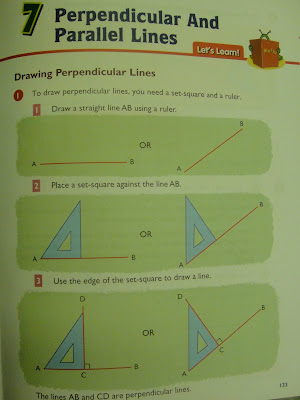## Saturday, April 3, 2010

### Teaching Perpendicular LinesQuestion: Should I get students to use set square to draw perpendicular lines in the first lesson or should I give them non-standard tools such as a right-angled triangle?

Hazel, a pre-service teacher in Singapore

This chapter comes after the chapter on angles where students have learnt about right angles. I think before students are taught how to draw perpendicular lines, they need to know what are perpendicular lines so that they are able to check if two lines are perpendicular. I am like to have given them two intersecting lines as well as lines joint at a point and ask them to measure the angles between the lines and to say that those that meet / intersect at right angles are called perpendicular lines. In my opinion, the chapter in the textbook starts too abruptly to get students to draw perpendicular lines without actually being taught what perpendicular lines are.

I like Hazel's idea of introducing other non-standard tools that can be used to draw perpendicular lines. I think a good lesson could involve the students being given some common objects such as ruler, protractor, set squares, triangle tangram set, an index card, ice-cream sticks and asked which of these they can use to draw lines that are perpendicular to each other.

The lesson can conclude with the whole class checking responses that they have generated using the criteria they have learnt.